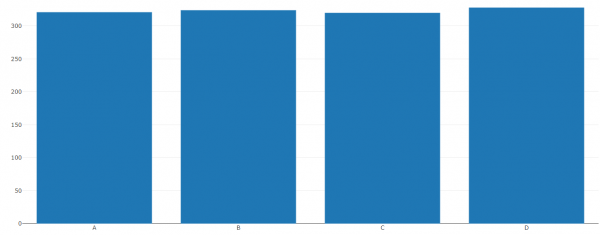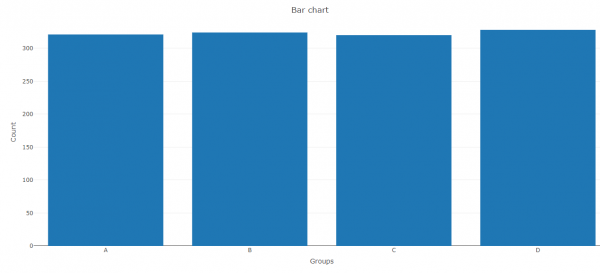# How to create a bar chart using plotly in R?

Plotly in R is a package specifically designed to create highly-interactive and publication-quality charts. The chart can be created by using plot_ly function of the package and there are three main arguments of plot_ly defined as x, y, and type, where x refers to the X-axis, y refers to the Y-axis and type refers to the chart type but the axes values are stored in a data frame or itself a shared.

## Example

> library(plotly)

Consider the below data frame:

Live Demo

> x<-c("A","B","C","D")
> count<-c(321,324,320,328)
> df<-data.frame(x,count)
> df

## Output

x count
1 A 321
2 B 324
3 C 320
4 D 328

Creating the bar plot for x:

> plot_ly(x=x,y=count,type='bar')

## Output

Warning message:arrange_() is deprecated as of dplyr 0.7.0.
Please use arrange() instead.
See vignette('programming') for more help
This warning is displayed once every 8 hours.
Call lifecycle::last_warnings() to see where this warning was generated.

Here it is showing a warning but it is not related to our chart hence there is no problem in ignoring it.

## Output:If we want to create the chart with main title and axes titles then we need to store it in an object and then use the layout function as shown below:

## Example

> Bar<-plot_ly(x=x,y=count,type='bar')
> layout(Bar,title="Bar chart",xaxis=list(title="Groups"),yaxis=list(title="Count"))

## Output: